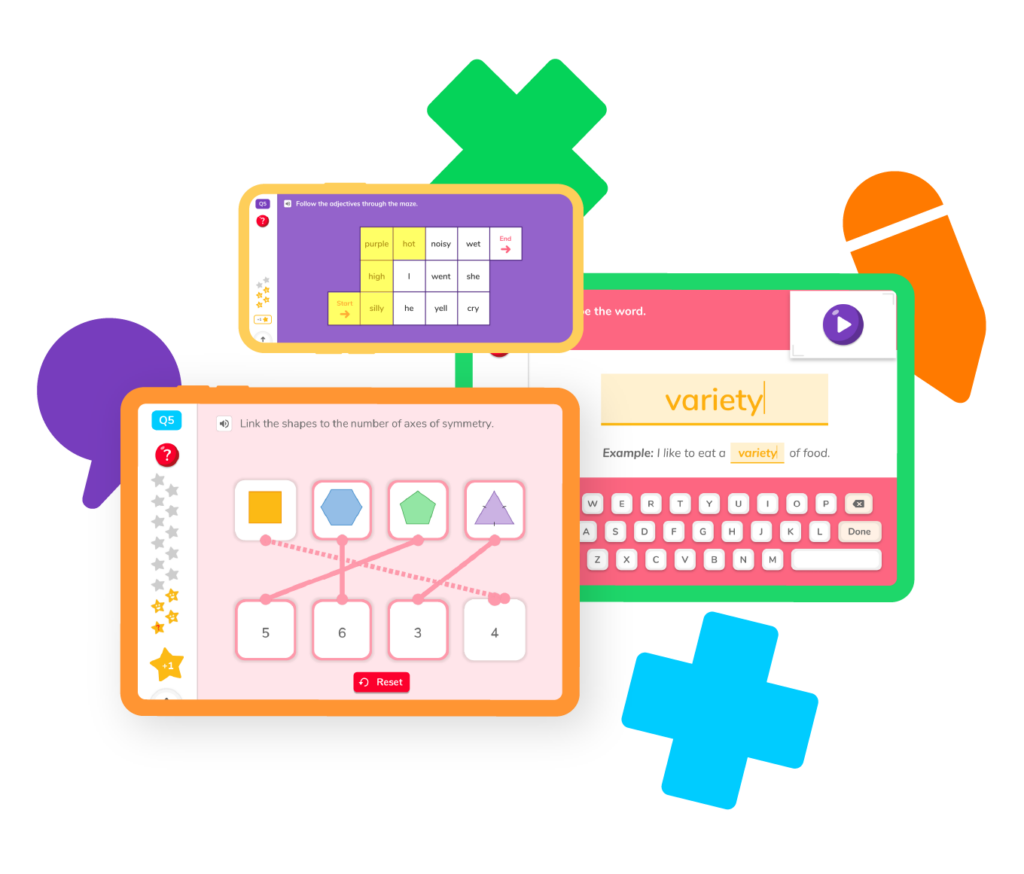# Learning Fractions

Ever wondered what it means when mom says, “we need to leave in half an hour?” Or how to add funky numbers like ⅙, ¼, and ⅘ together to get a big, whole number? And what is a whole number anyway? Learn all about what fractions are and how to add and subtract them to make all new fractions!## Fractions resourcesWhen it comes to learning fractions, there are plenty of skills to master! Select the skill you’d like to review and practice and find all the resources you need to become a fractions champion.

## Whole numbers vs fractions

Learn what a fraction is and when to use them.

How to add fractions together to make all new numbers.

## Subtracting fractions

How to subtract fractions and make all new numbers.

## Multiplying fractions

How to multiply fractions to make bigger—or smaller!—numbers.

## Dividing fractions

How divide fractions to make smaller—or bigger!—numbers.

## Fraction games

Test your skills in a fresh, fun way with our fraction games.

## Whole numbers vs. fractions

Learn what a fraction is and when to use them.

How to add fractions together to make all new numbers.

## Subtracting fractions

How to subtract fractions and make all new numbers.

## Multiplying fractions

How to multiply fractions to make bigger—or smaller!—numbers.

## Dividing fractions

How divide fractions to make smaller—or bigger!—numbers.

## Fraction games

Test your skills in a fresh, fun way with our fraction games.We know learning fractions is complex, and it can obviously take time. We’ve provided a few frequently asked questions many students have when they start out learning about and working with fractions.

A fraction, very simply, is a part of a whole. So, let’s say you have a whole pizza. A fraction is a single slice of that whole pizza. The pizza itself is made up of 10 slices, and you enjoy 1 slice.

When you look at a fraction, you’re looking at two numbers. The number on the top of the fraction is the numerator – or the slice of pizza – and the number on the bottom of the fraction is the denominator, or all the slices of pizza available.

Typically, students begin to learn about fractions in 1st grade. 1st graders will learn fractions, but only basic ones like ½, ⅓, and ¼. They will typically learn how to recognize fractions by dividing shapes into wholes, halves, thirds, and fourths. As they advance through 2nd, 3rd, 4th, and 5th grade, their lessons around fractions will become more complex, until they are multiplying and dividing fractions on their own.

There are several methods you can use, and it’s always best to talk to your student’s teacher to see if there are ways to support the learning that is already happening in the classroom. However, a good rule of thumb is to make learning fractions visual whenever possible. Folding paper, cutting circles into halves and fourths, and other similar strategies can be very effective.

Simplified fractions are fractions that have been reduced to their simplest, lowest form. For example, 4/16 can be simplified to 1/4.

Want to see that in even more detail. Let’s consider 5/15.

Obviously, 5 is divisible by 5, right? So 5÷5 = 1

And 15 is also divisible by 5. So 15÷5 = 3.

That means 5/15 can be simplified to 1/3.# Are you a parent, teacher or student?

Are you a parent or teacher?

## Hi there!

Book a chat with our team